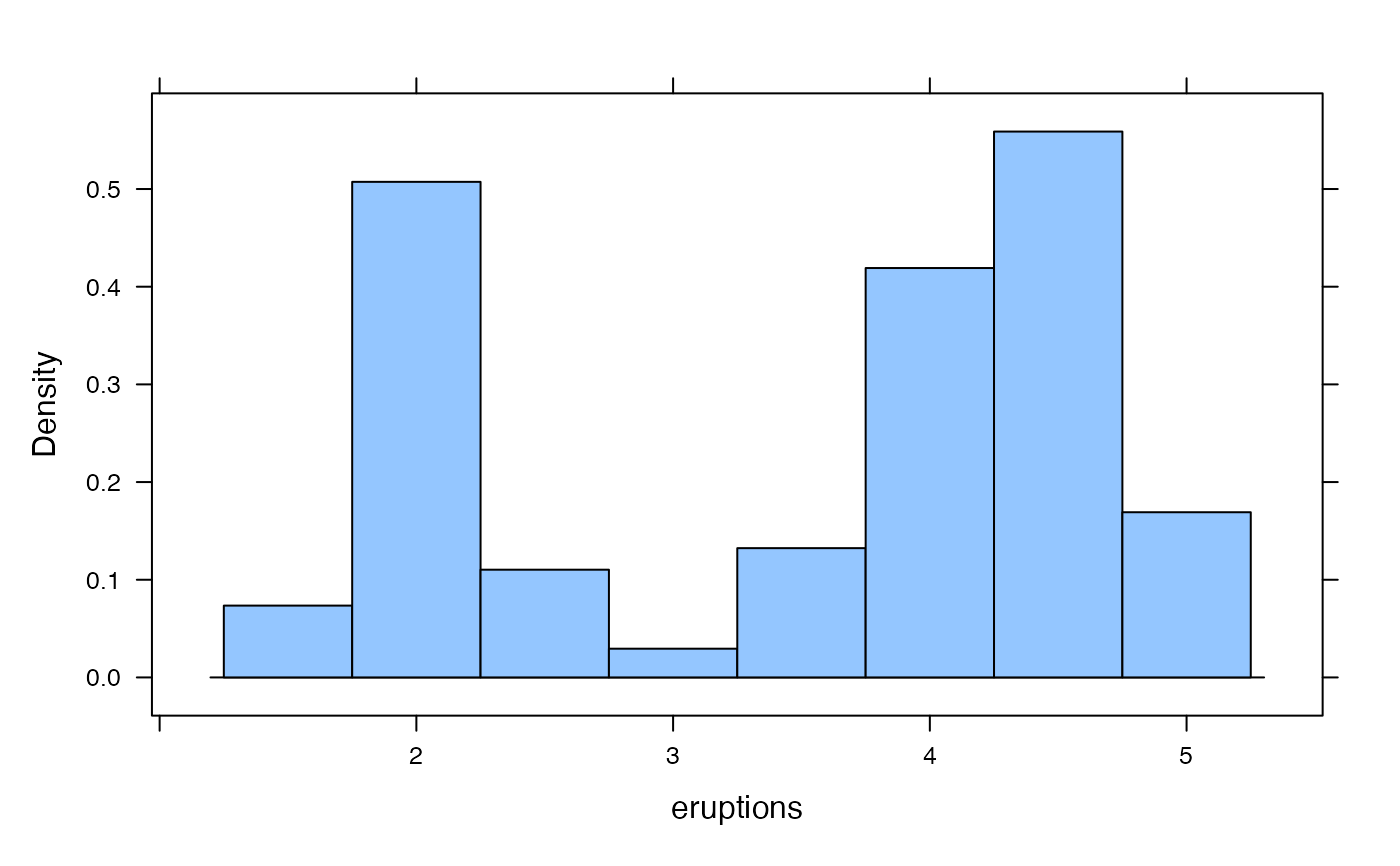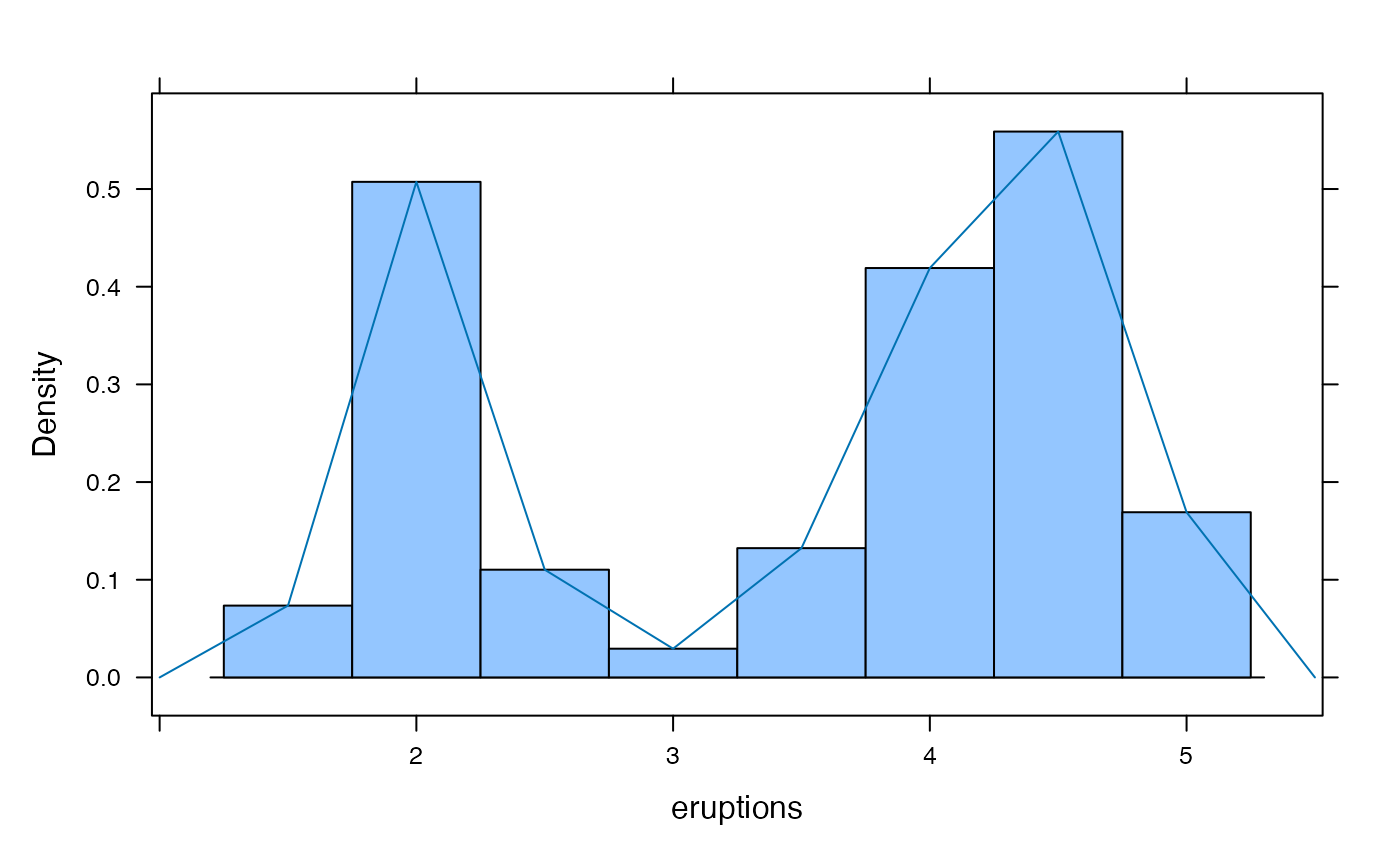Frequency polygons are an alternative to histograms that make it simpler to overlay multiple distributions.

freqpolygon(
x,
...,
panel = "panel.freqpolygon",
prepanel = "prepanel.default.freqpolygon"
)

prepanel.default.freqpolygon(
x,
darg = list(),
plot.points = FALSE,
ref = FALSE,
groups = NULL,
subscripts = TRUE,
jitter.amount = 0.01 * diff(current.panel.limits()$ylim), center = NULL, nint = NULL, breaks = NULL, width = darg$width,
type = "density",
...
)

panel.freqpolygon(
x,
darg = list(),
plot.points = FALSE,
ref = FALSE,
groups = NULL,
weights = NULL,
jitter.amount = 0.01 * diff(current.panel.limits()$ylim), type = "density", breaks = NULL, nint = NULL, center = NULL, width = darg$width,
gcol = trellis.par.get("reference.line")$col, glwd = trellis.par.get("reference.line")$lwd,
h,
v,
...,
identifier = "freqpoly"
)

## Arguments

x

a formula or a numeric vector

...

additional arguments passed on to histogram() and panel.

panel

a panel function

prepanel

a prepanel function

darg

a list of arguments for the function computing the frequency polygon. This exists primarily for compatibility with densityplot and is unlikely to be needed by the end user.

plot.points

one of TRUE, FALSE, "jitter", or "rug" indicating how points are to be displayed

ref

a logical indicating whether a horizontal reference line should be added (roughly equivalent to h=0)

groups, weights, jitter.amount, identifier

as in densityplot() or histogram()

subscripts

as in other lattice prepanel functions

center

center of one of the bins

nint

an approximate number of bins for the frequency polygon

breaks

a vector of breaks for the frequency polygon bins

width

width of the bins

type

one of 'density', 'percent', or 'count'

gcol

color of guidelines

glwd

width of guidelines

h, v

a vector of values for additional horizontal and vertical lines

a trellis object

## Note

This function make use of histogram to determine overall layout. Often this works reasonably well but sometimes it does not. In particular, when groups is used to overlay multiple frequency polygons, there is often too little head room. In the latter cases, it may be necessary to use ylim to determine an appropriate viewing rectangle for the plot.

## Examples

freqpolygon(~age | substance, data=HELPrct, v=35)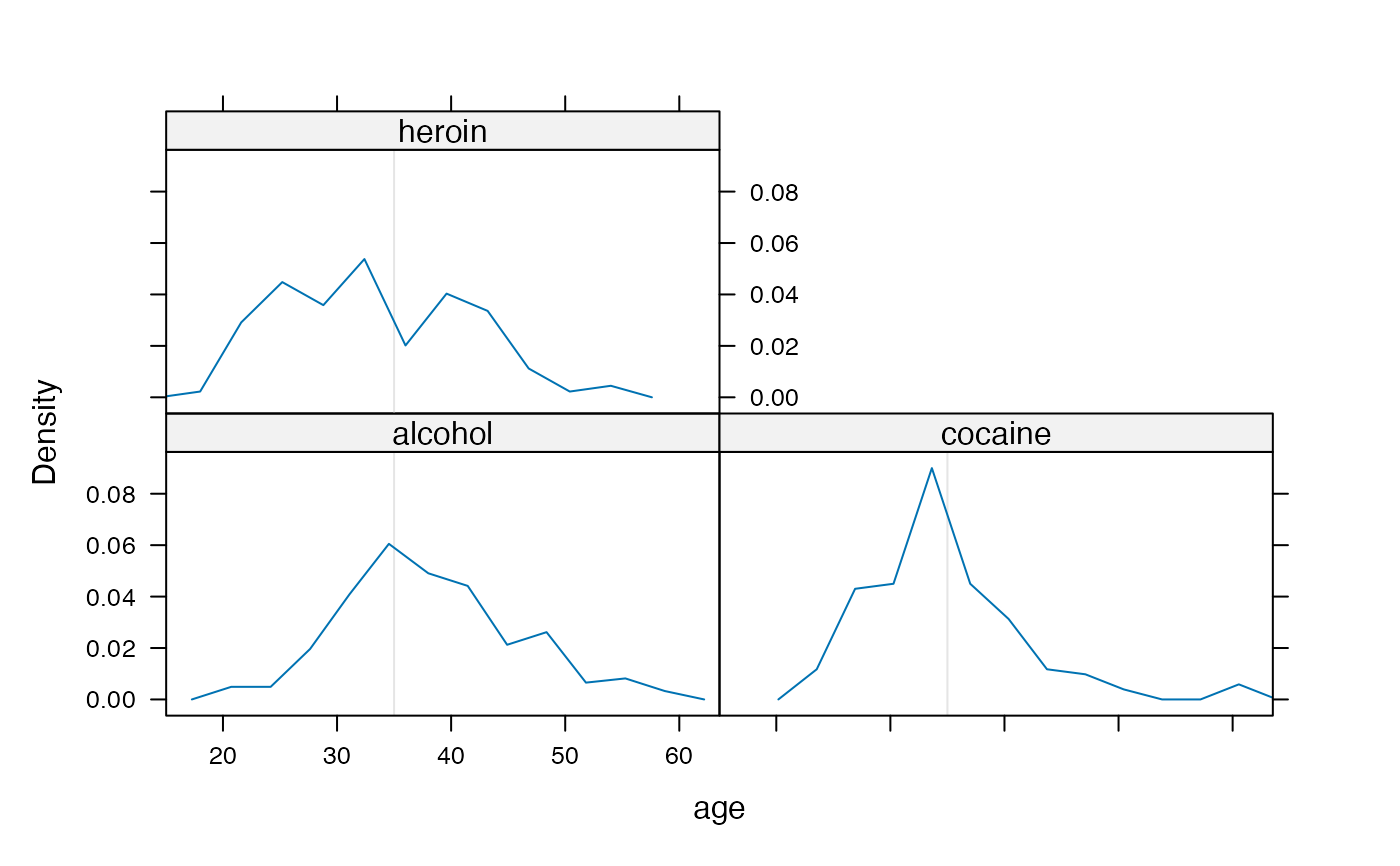freqpolygon(~age, data=HELPrct, labels=TRUE, type='count')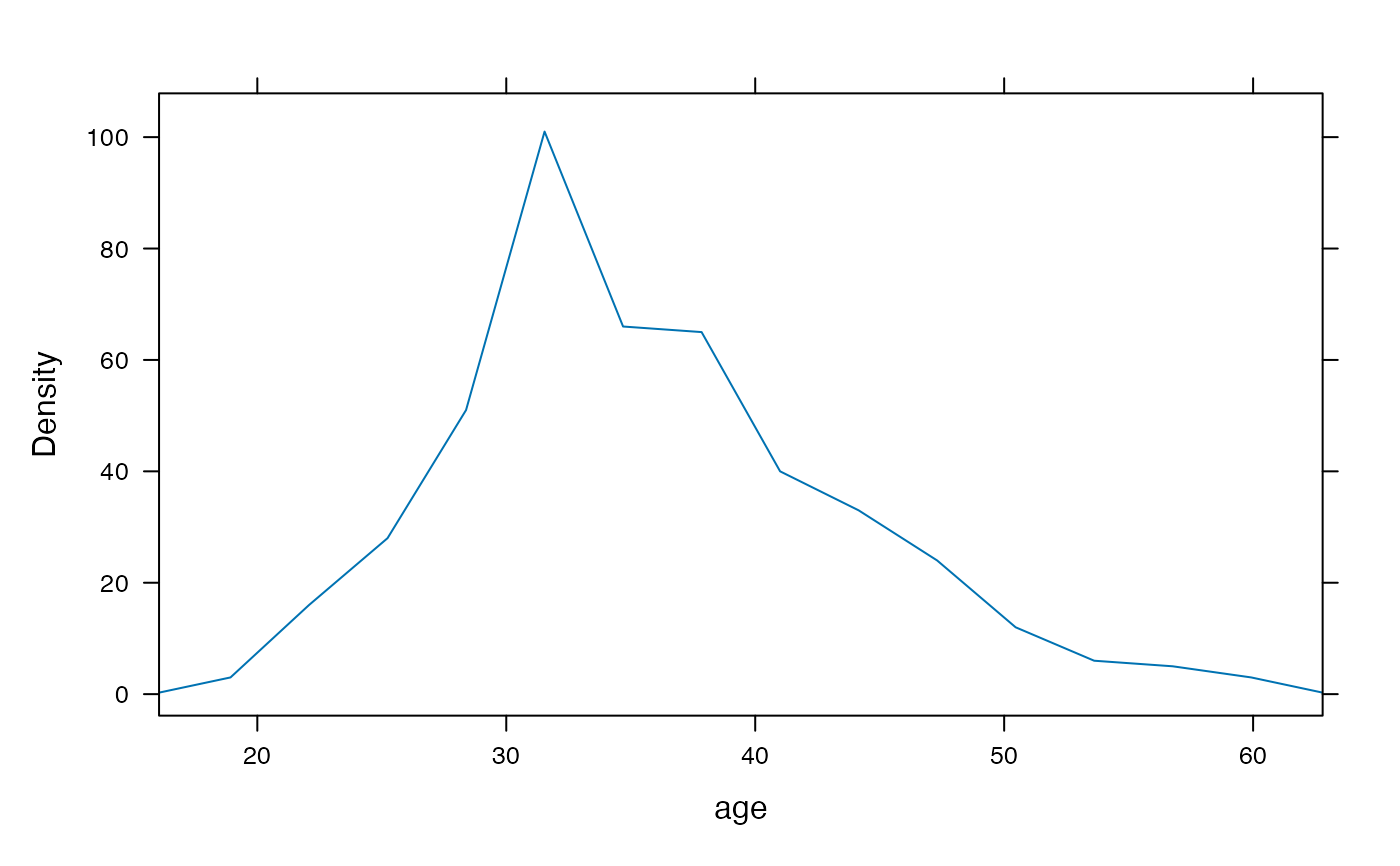freqpolygon(~age | substance, data=HELPrct, groups=sex)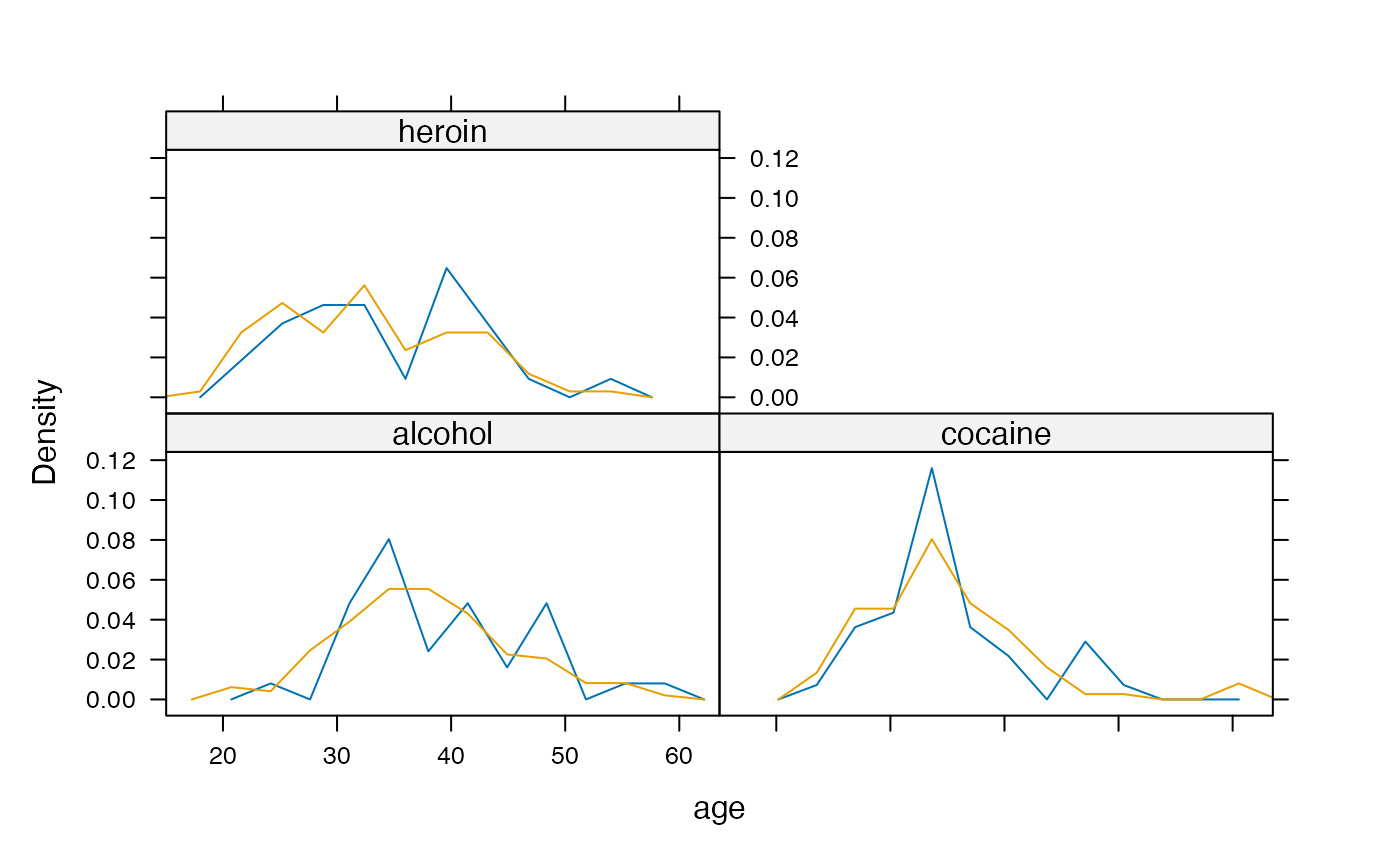freqpolygon(~age | substance, data=HELPrct, groups=sex, ylim=c(0,0.11))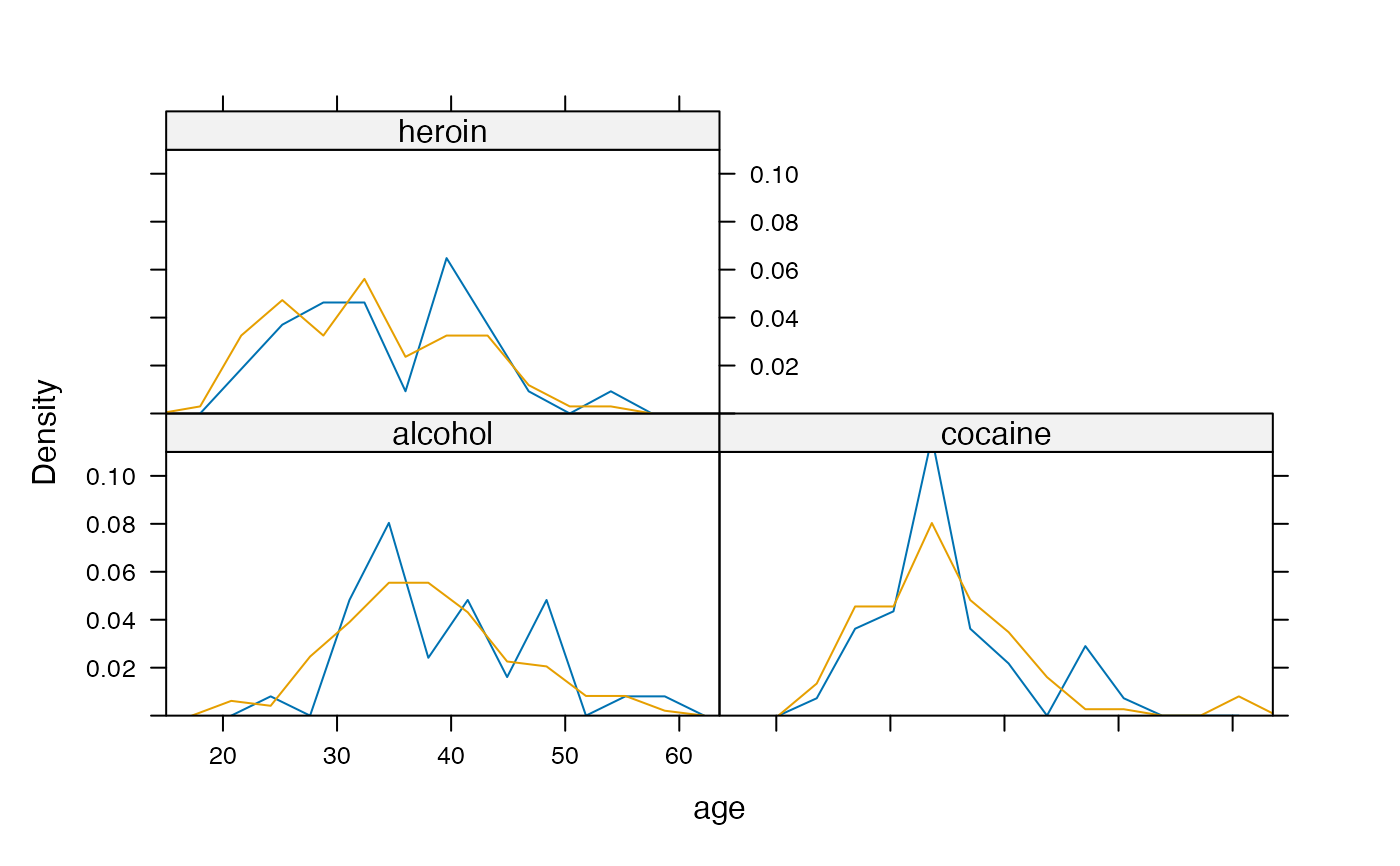## comparison of histogram and frequency polygon
histogram(~eruptions, faithful, type='density', width=.5)